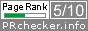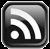# LEARN MS-ACCESS TIPS AND TRICKS

Learn Microsoft Access Advanced Programming Techniques, Tips and Tricks.

### Introduction.

There are times that a particular Class Module needs to be instantiated more than once, to use similar set of values for a particular Application.

For example: our ClsArea Class Module, we have designed for calculating carpet area of Rooms is a candidate for similar applications.  Assume that we would like to find out how many floor tiles we need to lay in a room then we should be able to input the Length and Width values of Tile also, besides the dimension values of Floor.  Since, both Floor and Tile have similar Property values as input we can use two instances of ClsArea Class Module, one instance for FLOOR area and the second instance for TILE area.  Floor-Area / Tile-Area gives the total number of tiles for a particular room.

### Two Instances of the same Class Module.

We can do this by creating two different instances of ClsArea Class Module in the Standard Module Program, if there is only one Room.

```Public Sub FloorTiles()
Dim FLOOR As ClsArea
Dim TILES As ClsArea
Dim flrArea As Double, tilearea As Double
Dim lngTiles As Long

Set FLOOR = New ClsArea
Set TILES = New ClsArea

FLOOR.strDesc = "Bed Room1"
FLOOR.dblLength = 25
FLOOR.dblWidth = 15
flrArea = FLOOR.Area()

TILES.strDesc = "Off-White"
TILES.dblLength = 2.5
TILES.dblWidth = 1.25
tilearea = TILES.Area()

lngTiles = flrArea / tilearea

Debug.Print FLOOR.strDesc & " Required Tiles: " & lngTiles & " Numbers - Color: " & TILES.strDesc

Set FLOOR = Nothing
Set TILES = Nothing

End Sub
```

### Handling Array of Objects.

But, what if we need to apply the above method for an array of several rooms with different sizes or color of tiles?  The answer to that is to create a new Class Module with two instances of the same ClsArea Class, one instance for Floor and the other for Tiles Properties.  Both instances are wrapped in a new Class Module.

Let us do that.

1. Open your Database and display Code Editing Window (ALT+F11).
2. Select Class Module from Insert Menu.
3. Change the Name Property value to ClsTiles.
4. Copy and Paste the following VBA Code into ClsTiles Class Module and save the Code:
```Option Compare Database
Option Explicit

Private pFLOOR As ClsArea
Private pTILES As ClsArea

Private Sub Class_Initialize()
Set pFLOOR = New ClsArea
Set pTILES = New ClsArea
End Sub

Private Sub Class_Terminate()
Set pFLOOR = Nothing
Set pTILES = Nothing
End Sub

Public Property Get Floor() As ClsArea
Set Floor = pFLOOR
End Property

Public Property Set Floor(ByRef NewValue As ClsArea)
Set pFLOOR = NewValue
End Property

Public Property Get Tiles() As ClsArea
Set Tiles = pTILES
End Property

Public Property Set Tiles(ByRef NewValue As ClsArea)
Set pTILES = NewValue
End Property

Public Function NoOfTiles() As Long
NoOfTiles = pFLOOR.Area() / pTILES.Area()
End Function
```

Both instances, pFLOOR and pTILES, are declared as Private Properties of ClsTiles Class Object.

The Class_Initialize() Subroutine instantiates both objects in memory when the ClsTiles Class Module is instantiated in the user program.

The Class_Terminate() subroutine removes both instances (pFLOOR and pTILES) from memory, when the ClsTiles Class Module instance is set to Nothing in the user program.

The Get and Set Property Procedures allows retrieval and assignment of values, from and to the pFLOOR instance respectively, in the ClsTiles Class Object.

The next Get and Set Property Procedure allows the same operations in the pTILES instance of the ClsArea Class.

We have added a new function NoOfTiles() in the new Class Module, to calculate the number of tiles, based on Floor-Area and Tile dimension.

Let us write a Program and learn the usage of multiple instances of the same Class Object,  in a new Class Module: ClsTiles.

5. Copy and paste the following VBA Code into a Standard Module:
```Public Sub TilesCalc()
Dim FTiles As ClsTiles
Dim TotalTiles As Long

Set FTiles = New ClsTiles

FTiles.Floor.strDesc = "Warehouse"
FTiles.Floor.dblLength = 100
FTiles.Floor.dblWidth = 50

FTiles.Tiles.dblLength = 2.5
FTiles.Tiles.dblWidth = 1.75

TotalTiles = FTiles.NoOfTiles()

Debug.Print "Site Name", "Floor Area", "Tile Area", "No. of Tiles"
Debug.Print FTiles.Floor.strDesc, FTiles.Floor.Area, FTiles.Tiles.Area, TotalTiles

End Sub
```
6. Keep the Debug Window Open (CTRL+G) to print the test data.
7. Click somewhere in the middle of the VBA Code and Press F5 Key to run the Code.  The result is printed on the Debug Window.

If you want to calculate the tile requirements of several rooms, or may be rooms of several floors of a high-rise building then you should run the above program that number of times and note down the values, which is practically very difficult.

### Finding Tile Requirement of Several Rooms.

Now, let us write another program to find the Tile requirements of several Rooms, with an  Array of ClsTiles Objects by inputting the Property values directly from keyboard.

8. Copy and Paste the following VBA Code into a Standard Module..
```Public Sub TilesCalc2()
Dim tmpFT As ClsTiles
Dim FTiles() As ClsTiles
Dim j As Long, L As Long, H As Long

For j = 1 To 3
Set tmpFT = New ClsTiles
'Floor dimension
With tmpFT.Floor
.strDesc = InputBox(Str(j) & ") Floor Desc", , 0)
.dblLength = InputBox(Str(j) & ") Floor Length", , 0)
.dblWidth = InputBox(Str(j) & ") Floor Width", , 0)
End With

'Tile Dimension
With tmpFT.Tiles
.strDesc = InputBox(Str(j) & ") Tiles Desc", , 0)
.dblLength = InputBox(Str(j) & ") Tile Length", , 0)
.dblWidth = InputBox(Str(j) & ") Tile Width", , 0)
End With

ReDim Preserve FTiles(1 To j) As ClsTiles
Set FTiles(j) = tmpFT

Set tmpFT = Nothing
Next

'Take Printout
L = LBound(FTiles)
H = UBound(FTiles)

Debug.Print "FLOOR", "Floor Area", "TILES", "Tile Area", "Total Tiles"
For j = L To H
With FTiles(j)
Debug.Print .Floor.strDesc, .Floor.Area(), .Tiles.strDesc, .Tiles.Area(), .NoOfTiles
End With
Next

'Remove all objects from memory
For j = L To H
Set FTiles(j) = Nothing
Next

End Sub

```
9. Keep the Debug Window open to print the output there.
10. Run the Code as before and input values for Floor and Tile dimensions for three Rooms.

As you can see from the above code that both values of Room and Tile dimensions goes into the same Class Object Array instance, side by side.

The above program is a demon that runs only for three set of values within the For. . . Next loop.  It can be modified with a conditional loop that runs for required number of times till a conditional break code terminates the program.

The program can be modified to save each set of data values and calculation results into a Table for future reference.

A Wrapper Class is a Container Class for instances of other Classes, Data Structures or instances collection of other objects.  Here we have used it to hold two instances of the same Class Object.

### List of All the Links on this Topic.

Share:

Comments subject to moderation before publishing.

## TranslateSubscribe in a readerYour email address:

Delivered by FeedBurner